BISMIIlAHIRAHMANIRAHIM DENGAN NAMA ALLAH YANG MAHA PERMURAH LAGI MAHA PENYAYANG, ASSALAMULAIKUM HELLO

ARIMA(p,d,q): ARIMA models are, in theory, the most general class of models for forecasting a time series which can be stationarized by transformations such as differencing and logging. In fact, the easiest way to think of ARIMA models is as fine-tuned versions of random-walk and random-trend models: the fine-tuning consists of adding lags of the differenced series and/or lags of the forecast errors to the prediction equation, as needed to remove any last traces of autocorrelation from the forecast errors.
The acronym ARIMA stands for "Auto-Regressive Integrated Moving Average." Lags of the differenced series appearing in the forecasting equation are called "auto-regressive" terms, lags of the forecast errors are called "moving average" terms, and a time series which needs to be differenced to be made stationary is said to be an "integrated" version of a stationary series. Random-walk and random-trend models, autoregressive models, and exponential smoothing models (i.e., exponential weighted moving averages) are all special cases of ARIMA models.
A nonseasonal ARIMA model is classified as an "ARIMA(p,d,q)" model, where:
• p is the number of autoregressive terms,
• d is the number of nonseasonal differences, and
• q is the number of lagged forecast errors in the prediction equation.
To identify the appropriate ARIMA model for a time series, you begin by identifying the order(s) of differencing needing to stationarize the series and remove the gross features of seasonality, perhaps in conjunction with a variance-stabilizing transformation such as logging or deflating. If you stop at this point and predict that the differenced series is constant, you have merely fitted a random walk or random trend model. (Recall that the random walk model predicts the first difference of the series to be constant, the seasonal random walk model predicts the seasonal difference to be constant, and the seasonal random trend model predicts the first difference of the seasonal difference to be constant--usually zero.) However, the best random walk or random trend model may still have autocorrelated errors, suggesting that additional factors of some kind are needed in the prediction equation.

ARIMA(0,1,0) = random walk: In models we have studied previously, we have encountered two strategies for eliminating autocorrelation in forecast errors. One approach, which we first used in regression analysis, was the addition of lags of the stationarized series. For example, suppose we initially fit the random-walk-with-growth model to the time series Y. The prediction equation for this model can be written as:...where the constant term (here denoted by "mu") is the average difference in Y. This can be considered as a degenerate regression model in which DIFF(Y) is the dependent variable and there are no independent variables other than the constant term. Since it includes (only) a nonseasonal difference and a constant term, it is classified as an "ARIMA(0,1,0) model with constant." Of course, the random walk without growth would be just an ARIMA(0,1,0) model without constant

ARIMA(1,1,0) = differenced first-order autoregressive model: If the errors of the random walk model are autocorrelated, perhaps the problem can be fixed by adding one lag of the dependent variable to the prediction equation--i.e., by regressing DIFF(Y) on itself lagged by one period. This would yield the following prediction equation: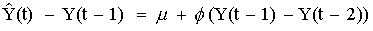which can be rearranged to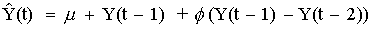This is a first-order autoregressive, or "AR(1)", model with one order of nonseasonal differencing and a constant term--i.e., an "ARIMA(1,1,0) model with constant." Here, the constant term is denoted by "mu" and the autoregressive coefficient is denoted by "phi", in keeping with the terminology for ARIMA models popularized by Box and Jenkins. (In the output of the Forecasting procedure in Statgraphics, this coefficient is simply denoted as the AR(1) coefficient.)

ARIMA(0,1,1) without constant = simple exponential smoothing: Another strategy for correcting autocorrelated errors in a random walk model is suggested by the simple exponential smoothing model. Recall that for some nonstationary time series (e.g., one that exhibits noisy fluctuations around a slowly-varying mean), the random walk model does not perform as well as a moving average of past values. In other words, rather than taking the most recent observation as the forecast of the next observation, it is better to use an average of the last few observations in order to filter out the noise and more accurately estimate the local mean. The simple exponential smoothing model uses an exponentially weighted moving average of past values to achieve this effect. The prediction equation for the simple exponential smoothing model can be written in a number of mathematically equivalent ways, one of which is: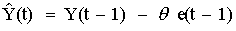...where e(t-1) denotes the error at period t-1. Note that this resembles the prediction equation for the ARIMA(1,1,0) model, except that instead of a multiple of the lagged difference it includes a multiple of the lagged forecast error. (It also does not include a constant term--yet.) The coefficient of the lagged forecast error is denoted by the Greek letter "theta" (again following Box and Jenkins) and it is conventionally written with a negative sign for reasons of mathematical symmetry. "Theta" in this equation corresponds to the quantity "1-minus-alpha" in the exponential smoothing formulas we studied earlier.
When a lagged forecast error is included in the prediction equation as shown above, it is referred to as a "moving average" (MA) term. The simple exponential smoothing model is therefore a first-order moving average ("MA(1)") model with one order of nonseasonal differencing and no constant term --i.e., an "ARIMA(0,1,1) model without constant." This means that in Statgraphics (or any other statistical software that supports ARIMA models) you can actually fit a simple exponential smoothing by specifying it as an ARIMA(0,1,1) model without constant, and the estimated MA(1) coefficient corresponds to "1-minus-alpha" in the SES formula.

ARIMA(0,1,1) with constant = simple exponential smoothing with growth: By implementing the SES model as an ARIMA model, you actually gain some flexibility. First of all, the estimated MA(1) coefficient is allowed to be negative: this corresponds to a smoothing factor larger than 1 in an SES model, which is usually not allowed by the SES model-fitting procedure. Second, you have the option of including a constant term in the ARIMA model if you wish, in order to estimate an average non-zero trend. The ARIMA(0,1,1) model with constant has the prediction equation: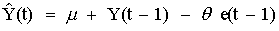The one-period-ahead forecasts from this model are qualitatively similar to those of the SES model, except that the trajectory of the long-term forecasts is typically a sloping line (whose slope is equal to mu) rather than a horizontal line.

ARIMA(0,2,1) or (0,2,2) without constant = linear exponential smoothing: Linear exponential smoothing models are ARIMA models which use two nonseasonal differences in conjunction with MA terms. The second difference of a series Y is not simply the difference between Y and itself lagged by two periods, but rather it is the first difference of the first difference--i.e., the change-in-the-change of Y at period t. Thus, the second difference of Y at period t is equal to (Y(t)-Y(t-1)) - (Y(t-1)-Y(t-2)) = Y(t) - 2Y(t-1) + Y(t-2). A second difference of a discrete function is analogous to a second derivative of a continuous function: it measures the "acceleration" or "curvature" in the function at a given point in time.
The ARIMA(0,2,2) model without constant predicts that the second difference of the series equals a linear function of the last two forecast errors: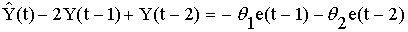which can be rearranged as: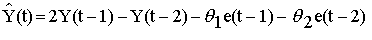where theta-1 and theta-2 are the MA(1) and MA(2) coefficients. This is essentially the same as Brown's linear exponential smoothing model, with the MA(1) coefficient corresponding to the quantity 2*(1-alpha) in the LES model. To see this connection, recall that forecasting equation for the LES model is: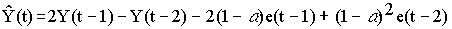Upon comparing terms, we see that the MA(1) coefficient corresponds to the quantity 2*(1-alpha) and the MA(2) coefficient corresponds to the quantity -(1-alpha)^2 (i.e., "minus (1-alpha) squared"). If alpha is larger than 0.7, the corresponding MA(2) term would be less than 0.09, which might not be significantly different from zero, in which case an ARIMA(0,2,1) model probably would be identified.

A "mixed" model--ARIMA(1,1,1): The features of autoregressive and moving average models can be "mixed" in the same model. For example, an ARIMA(1,1,1) model with constant would have the prediction equation: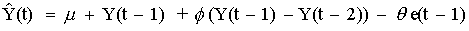Normally, though, we will try to stick to "unmixed" models with either only-AR or only-MA terms, because including both kinds of terms in the same model sometimes leads to overfitting of the data and non-uniqueness of the coefficients.